Home > CC2MN > Chapter cc29 > Lesson cc29.2.2 > Problem9-66

9-66.
1. Use the rectangular prism shown at right to complete parts (a) through (d) below. Homework Help ✎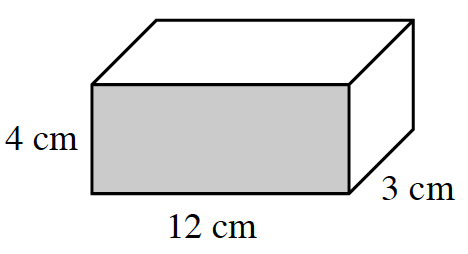1. Calculate the surface area of the prism. Show all of your subproblems.

2. Explain why the exponent for area units is 2 (that is, cm2).

3. Calculate the volume of the prism.

4. Explain why the exponent for volume units is a 3 (that is, cm3).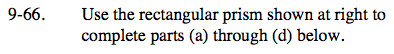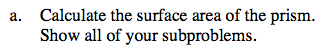To find the surface area, find the area of each face of the prism.

There are 6 faces in a rectangular prism.
Each of the three different faces shown in the image have an opposite face, so find the area of each of those 3 faces and multiply it by 2.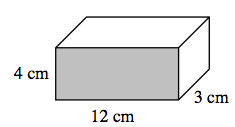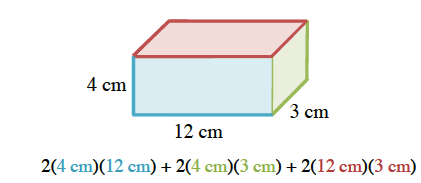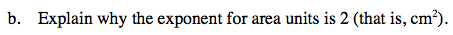How many terms do you multiply together to find area?

To find area, you multiply two dimensions.
Just as (x)(x) = x2, (cm)(cm) = cm².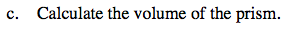To find the volume of a rectangular prism, multiply the base, height, and width together.

Volume = (12 cm)(4 cm)(3 cm)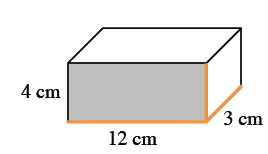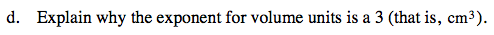See part (b).